# Box plot and Histogram exploration on Iris data

```Attribute Information:
-> sepal length in cm
-> sepal width in cm
-> petal length in cm
-> petal width in cm
-> class:
Iris Setosa
Iris Versicolour
Iris Virginica

Number of Instances: 150

Summary Statistics:
Min  Max   Mean    SD   Class Correlation
sepal length: 4.3  7.9   5.84  0.83    0.7826
sepal width: 2.0  4.4   3.05  0.43   -0.4194
petal length: 1.0  6.9   3.76  1.76    0.9490  (high!)
petal width: 0.1  2.5   1.20  0.76    0.9565  (high!)

Class Distribution: 33.3% for each of 3 classes.
```

 `import` `numpy as np ` `import` `pandas as pd ` `import` `matplotlib.pyplot as plt `

 `data ``=` `pd.read_csv(``"Iris.csv"``) ` ` `  `print` `(data.head(``10``)) `

Output: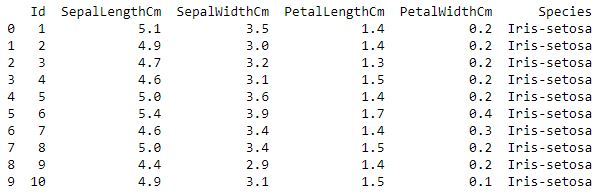Description

 `data.describe() `

Output: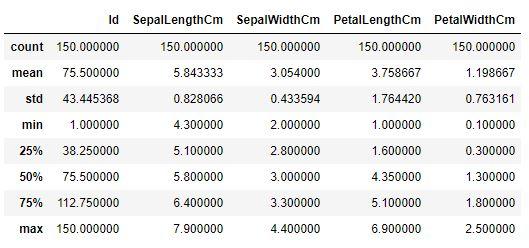Info

 `data.info() `

Output: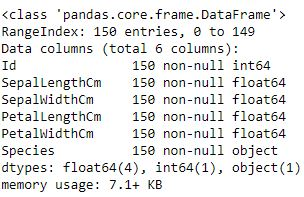Code #1: Histogram for Sepal Length

 `plt.figure(figsize ``=` `(``10``, ``7``)) ` `x ``=` `data[``"SepalLengthCm"``] ` ` `  `plt.hist(x, bins ``=` `20``, color ``=` `"green"``) ` `plt.title(``"Sepal Length in cm"``) ` `plt.xlabel(``"Sepal_Length_cm"``) ` `plt.ylabel(``"Count"``) `

Output: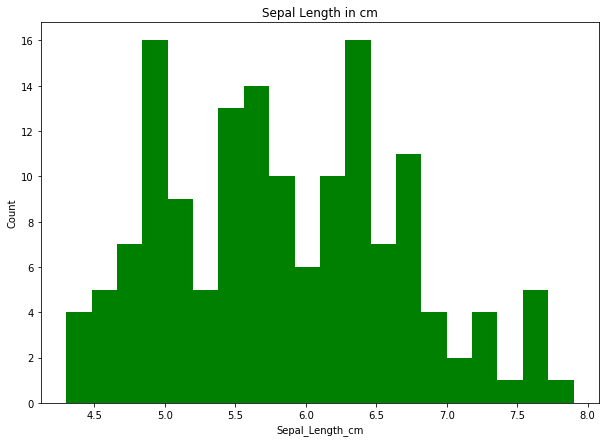Code #2: Histogram for Sepal Width

 `plt.figure(figsize ``=` `(``10``, ``7``)) ` `x ``=` `data.SepalWidthCm ` ` `  `plt.hist(x, bins ``=` `20``, color ``=` `"green"``) ` `plt.title(``"Sepal Width in cm"``) ` `plt.xlabel(``"Sepal_Width_cm"``) ` `plt.ylabel(``"Count"``) ` ` `  `plt.show() `

Output: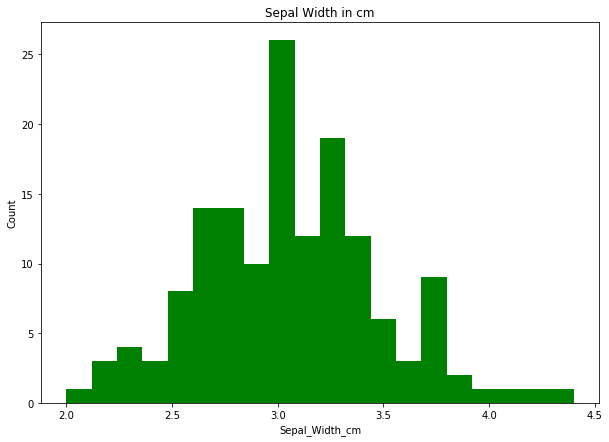Code #3: Histogram for Petal Length

 `plt.figure(figsize ``=` `(``10``, ``7``)) ` `x ``=` `data.PetalLengthCm ` ` `  `plt.hist(x, bins ``=` `20``, color ``=` `"green"``) ` `plt.title(``"Petal Length in cm"``) ` `plt.xlabel(``"Petal_Length_cm"``) ` `plt.ylabel(``"Count"``) ` ` `  `plt.show() `

Output: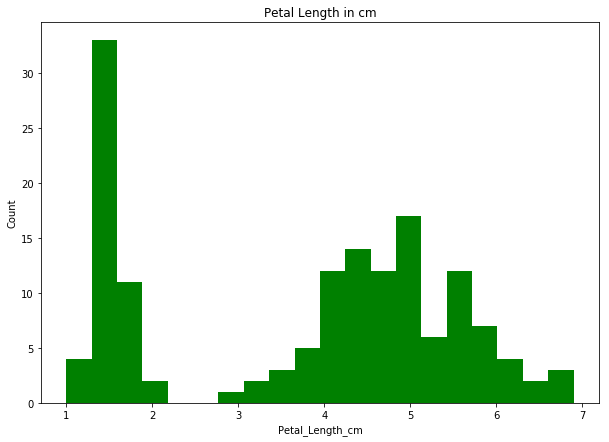Code #4: Histogram for Petal Width

 `plt.figure(figsize ``=` `(``10``, ``7``)) ` `x ``=` `data.PetalWidthCm ` ` `  `plt.hist(x, bins ``=` `20``, color ``=` `"green"``) ` `plt.title(``"Petal Width in cm"``) ` `plt.xlabel(``"Petal_Width_cm"``) ` `plt.ylabel(``"Count"``) ` ` `  `plt.show() `

Output: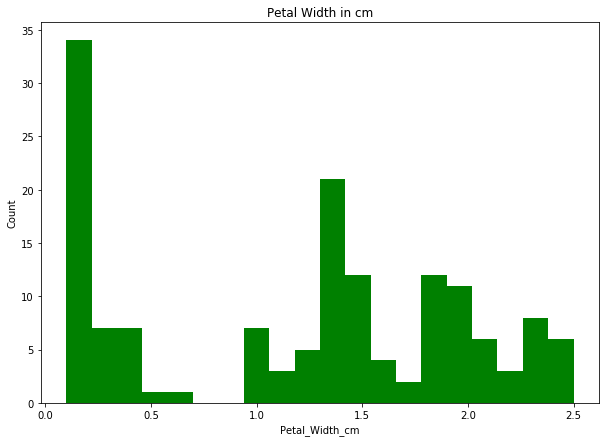Code #5: Data preparation for Box Plot

 `# removing Id column ` `new_data ``=` `data[[``"SepalLengthCm"``, ``"SepalWidthCm"``, ``"PetalLengthCm"``, ``"PetalWidthCm"``]] ` `print``(new_data.head()) `

Output :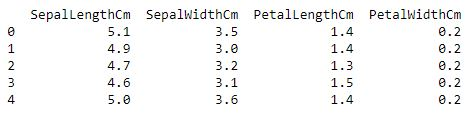Code #6: Box Plot for Iris Data

 `plt.figure(figsize ``=` `(``10``, ``7``)) ` `new_data.boxplot() `

Output :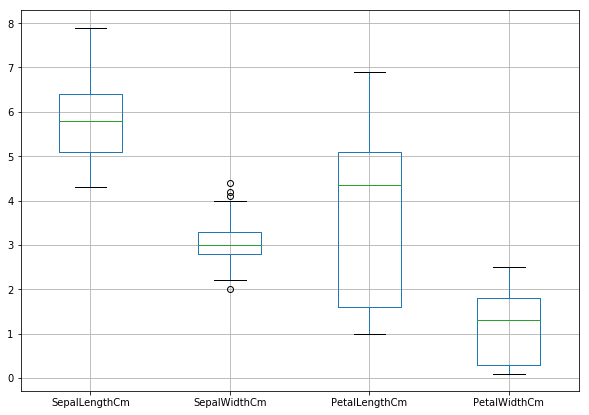Attention geek! Strengthen your foundations with the Python Programming Foundation Course and learn the basics.

To begin with, your interview preparations Enhance your Data Structures concepts with the Python DS Course.

My Personal Notes arrow_drop_upAspire to Inspire before I expire

If you like GeeksforGeeks and would like to contribute, you can also write an article using contribute.geeksforgeeks.org or mail your article to contribute@geeksforgeeks.org. See your article appearing on the GeeksforGeeks main page and help other Geeks.

Please Improve this article if you find anything incorrect by clicking on the "Improve Article" button below.

Improved By : ManasChhabra2

Article Tags :
Practice Tags :

Be the First to upvote.

Please write to us at contribute@geeksforgeeks.org to report any issue with the above content.